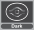Template:CM card/doc

{{CM card}} is used to create tables for monsters from the Yu-Gi-Oh! Capsule Monsters Collectible Figure Game.

Usage

{{CM card
| name           =
| faction        =
| monster_cost   =
| hp             =
| dv             =
| mp             =
| ap             =
| move_cost      =
| move_pattern   =
| move_range     =
| attack_cost    =
| attack_pattern =
| attack_range   =
| lore           =
| number         =
}}

Examples

{{CM card
| faction        = Light
| monster_cost   = 4
| hp             = 7
| dv             = 3
| move_cost      = 1
| move_pattern   = +
| move_range     = 4
| attack_cost    = 1
| attack_pattern = +
| attack_range   = 1
| lore           = FLYING
| number         = 124
}}
{{CM card
| faction        = Light
| monster_cost   = 5
| hp             = 7
| dv             = 3
| move_cost      = 1
| move_pattern   = +
| move_range     = 4
| attack_cost    = 1
| attack_pattern = +
| attack_range   = 1
| lore           = Blue-Eyes White Dragon (2) can fly and move over apposing monsters in its path.<br />When Blue-Eyes White Dragon (2) attacks add +1 Damage for each Monster (including this Monster) that you have in the Coliseum.
| number         = 125
}}
Blue-Eyes White Dragon (2)
{{CM card
| faction        = Dark
| mp             = 10/19
| ap             = 5/7
| move_cost      = 1
| move_pattern   = *
| move_range     = 1
| lore           = At the start of your turn, if you have any monsters on your opponent's side of the Coliseum, put one Damage Marker on your opponent's King.
| number         = 101
}}
Dark King
FactionDark 10/19 5/7 At the start of your turn, if you have any monsters on your opponent's side of the Coliseum, put one Damage Marker on your opponent's King.